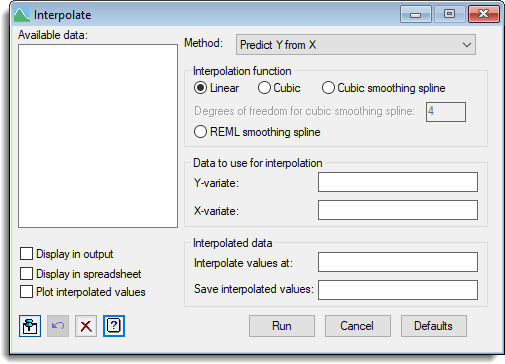1. Home
2. Interpolation

# Interpolation

Interpolation fits a piecewise line or curve through a set of points, then predicts values at intermediate values of x or y using the fitted curve. The values to interpolate must be at points within the range of the given x or y data. If the interpolating curve is Y=F(X), then for a given x, we obtain the interpolated value y = F(x). For inverse interpolation, for a given y, x is found, such that y = F(x), if possible. Two smoothing functions are provided. These do not give a curve that passes through every point, but smooth out random variation around a smooth trend which best fits the data for a given degree of flexibility. This allows predictions to be estimated with reduction in the random variation from point to point.

Data | Interpolation.## Available data

Lists variates that are currently available. Double-click a name to copy it into the edit field or type the name.

## Method

This defines the type of interpolation.

 Predict Y from X given the x-values, predict the y-values by interpolation. Predict X from Y given the y-values, predict the x-values by inverse interpolation. Predict missing values in both Y and X Predict values for the missing observations within both the x and y variates. If a y-value is missing the corresponding x-value is used to interpolate the missing y-value, and vice-versa for missing x-values.

## Interpolation function

This defines the type of curve fitted to the points in the original series.

 Linear a straight line joins the points in a piecewise linear function. Cubic a cubic polynomial is fitted through the 4 points around each interval. Cubic smoothing spline a cubic smoothing spline with the specified degrees of freedom is fitted to the series using the FIT command. REML smoothing spline a REML smoothing spline is fitted to the points, where the amount of smoothing is estimated as a variance component.

## Data to use for interpolation

These two fields define the points in the series being interpolated from.

 Y-variate variate containing the y-values in the data. X-variate variate containing the x-values in the data.

## Interpolated data

Provides fields to specify the values to interpolate at and save the resulting values. These fields are only available when either the Predict Y from X or Predict X from Y methods are selected. The values to interpolate at can be supplied within a variate, as an individual number or a series of numbers separated by spaces or commas (e.g. 1 3.5 5.8 or 1,3.5,5.8).

 Interpolate values at contains the known x- or y-values where the y- or x-values will be interpolated at. Save interpolated values a name of a variate to save the interpolated values.

## Display in output

When selected, the interpolated values will be displayed in the Output window.